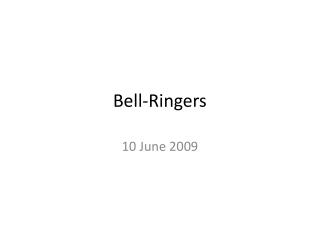DownloadDownload PresentationBell-Ringers

# Bell-Ringers

Download Presentation## Bell-Ringers

- - - - - - - - - - - - - - - - - - - - - - - - - - - E N D - - - - - - - - - - - - - - - - - - - - - - - - - - -
##### Presentation Transcript

1. Bell-Ringers 10 June 2009

2. Bell-Ringer • 2nd pd, 10 June 2009 • LT: Describe fundamental properties of matter (mass, weight, volume, density). Galileo’s Thermometer shows the temperature using floating objects. The lowest floating object shows the temperature. Explain how you think this might work.

3. Two tools are most often used to measure the MASS (amount of matter) and WEIGHT (gravitational force).

4. Mass: Measured in kilograms (kg) with a BALANCE. Weight: Measured in newtons (N) with a spring SCALE.

5. Mass: NOT affected by gravity. Weight: AFFECTED by gravity.

6. Mass, Volume, & Density • Mass • Amount of matter in an object (kg or g) • 1 small paperclip has a mass of about 1 gram • Volume • Amount of space an object takes up (L or mL) • 20 drops of water take up 1 mL (=1 cc) of volume • Density • How tightly packed something is • The density of water is about 1 gm/mL

7. Mass • Mass is the amount of material in the toy box.

8. Volume • Volume is how much space the toy box contains.

9. Density • Density is how tightly things are packed. • Can you think of things that are high density? • Can you think of things that are low density?

10. Summary • Mass is “so much matter” • Volume is “so much space” • Density is “so much matter contained in so much space”

11. Bell-Ringer • 3rd pd, 10 June 2009 • LT: Measure the mass, volume, and density of a material. Sociologists might speak about the population density of a community. What does this mean? How is this related to the density of a material?